#There are 366 different Starters of The Day, many to choose from. You will find below some starters on the topic of Factors. A lesson starter does not have to be on the same topic as the main part of the lesson or the topic of the previous lesson. It is often very useful to revise or explore other concepts by using a starter based on a totally different area of Mathematics.

Main Page

### Factors Starters:A game based around the concept of factors and abundant numbers.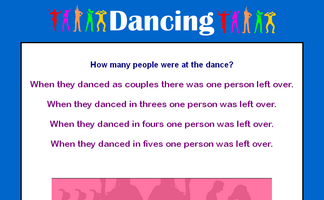Work out how many people were at the dance from the clues given.Spot the factors and the multiples amongst the numbers in the grid.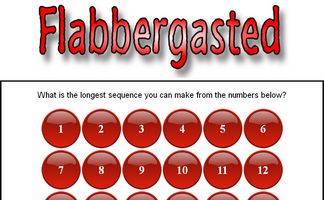If each number in a sequence must be a factor or multiple of the previous number what is the longest sequence that can be made from the given numbers?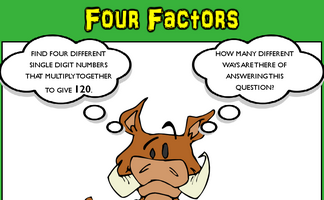Find four single digit numbers that multiply together to give 120. How many different ways are there of answering this question?A puzzle about the lifts in a hotel which serve floors based on the day of the week.Six is a perfect number as it is the sum of its factors. Can you find any other perfect numbers?The Verruca Value of a word is the number of vowels multiplied by the number of consonants. How many words can you find with Verruca Value of 24?

## Exercises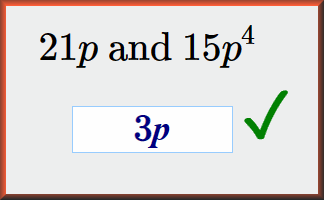#### Algebraic HCF

Exercises providing practice finding the highest common factor of algebraic expressions.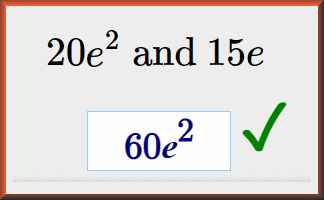#### Algebraic LCM

Exercises providing practice finding the lowest common multiple of algebraic expressions.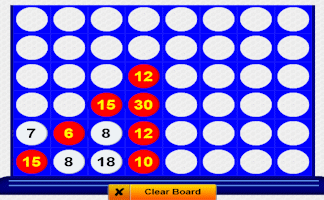#### Connect 4 Factors

This a game for one or two players. The winner is the first to line up four numbers with a common factor.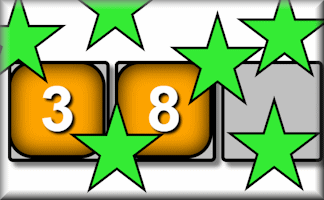#### Delightfully Divisible

Arrange the digits one to nine to make a number which is divisible in the way described.#### Divisibility Test

Practise using the quick ways to spot whether a number is divisible by the digits two to nine.#### Divisibility Tests 2-12

A visual aid designed to be projected in the classroom. Here you can find the quick ways of telling whether a number is exactly divisible by the numbers two to twelve.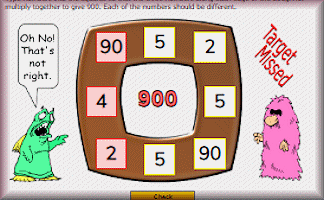#### Doughnut Dissection

A puzzle to find four different ways of making 900 by multiplying together three different numbers.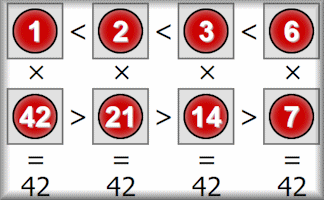#### Factor Pairs

Find the factor pairs of the given numbers and arrange them in order.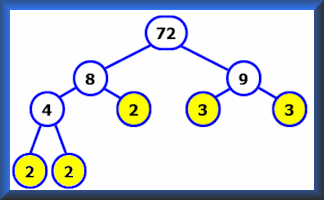#### Factor Trees

Create factor trees to find the prime factors of the given numbers.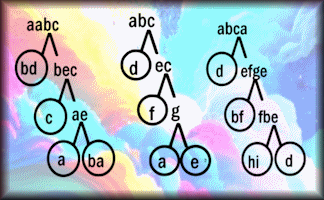#### Factor Trees Challenge

Can you determine the unique digits that will complete these factor trees?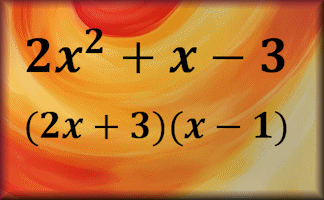#### Factorising

Practise the skills of algebraic factorisation in this structured online self marking exercise.#### Flabbergasted Game

This game for one or two players is an exciting challenge to demonstrate an understanding of factors and multiples.#### HCF and LCM

Practise finding the highest common factor (H.C.F), sometimes called the greatest common divisor, and the lowest common multiple (L.C.M) of two numbers.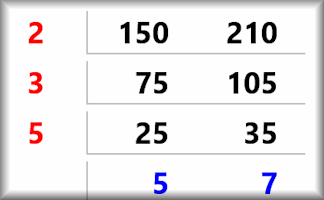#### HCF and LCM Calculator

A demonstration of how to find the highest common factor (HCF or GCD) and the lowest common multiple (LCM) of two numbers.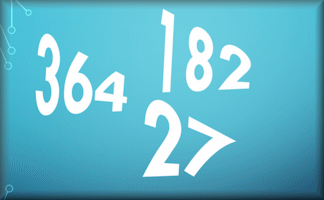#### How Many Factors?

Work out the number of factors a number has then write them all as a list.#### Number Grids

Investigate the properties of number with these interactive number grids.#### Pick The Primes

Pick the prime fruit from the tree as quickly as possible. Practise to improve your personal best time.#### Prime Labyrinth

Find the path to the centre of the labyrinth by moving along the prime numbers.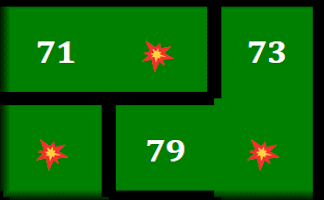#### Prime Numbers Jigsaw

Interactive jigsaw puzzles of different types of grids containing prime numbers.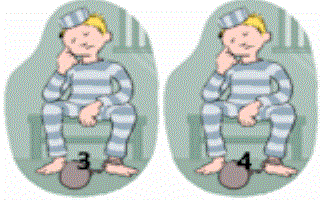#### Prison Cell Problem

A number patterns investigation involving prisoners and prison guards.#### Product Square

Arrange the given numbers in a three by three grid to obtain the diagonal, row and column products.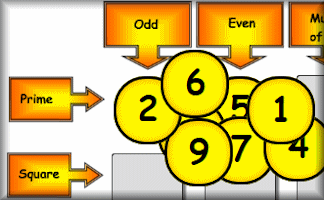#### Satisfy

Place the nine numbers in the table so they obey the row and column headings about the properties of the numbers.#### Scallywags and Scoundrels

Arrange the scallywags and scoundrels on the chairs so that the numbers of any two sitting next to each other add up to a prime number.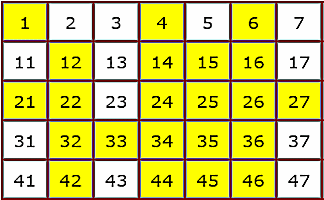#### Sieve of Eratosthenes

A self checking, interactive version of the Sieve of Eratosthenes method of finding prime numbers.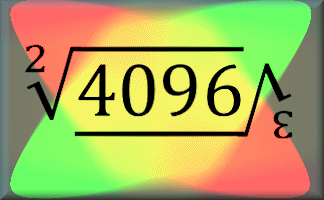#### Square and Cube Roots

Find square roots and cube roots by first calculating the prime factorisation of a number.#### Three Ways

Find three different ways of multiplying four different digits together to get the given target number. There are nine levels for this online challenge.#### Threes and Fives

A game for two players who take turns to add a domino to the line in order to score threes and fives.#### Times Square

Practise your times tables with this self-checking multiplication grid.

### Search

The activity you are looking for may have been classified in a different way from the way you were expecting. You can search the whole of Transum Maths by using the box below.

Have today's Starter of the Day as your default homepage. Copy the URL below then select
Tools > Internet Options (Internet Explorer) then paste the URL into the homepage field.

Set as your homepage (if you are using Internet Explorer)

Do you have any comments? It is always useful to receive feedback and helps make this free resource even more useful for those learning Mathematics anywhere in the world. Click here to enter your comments.For All: